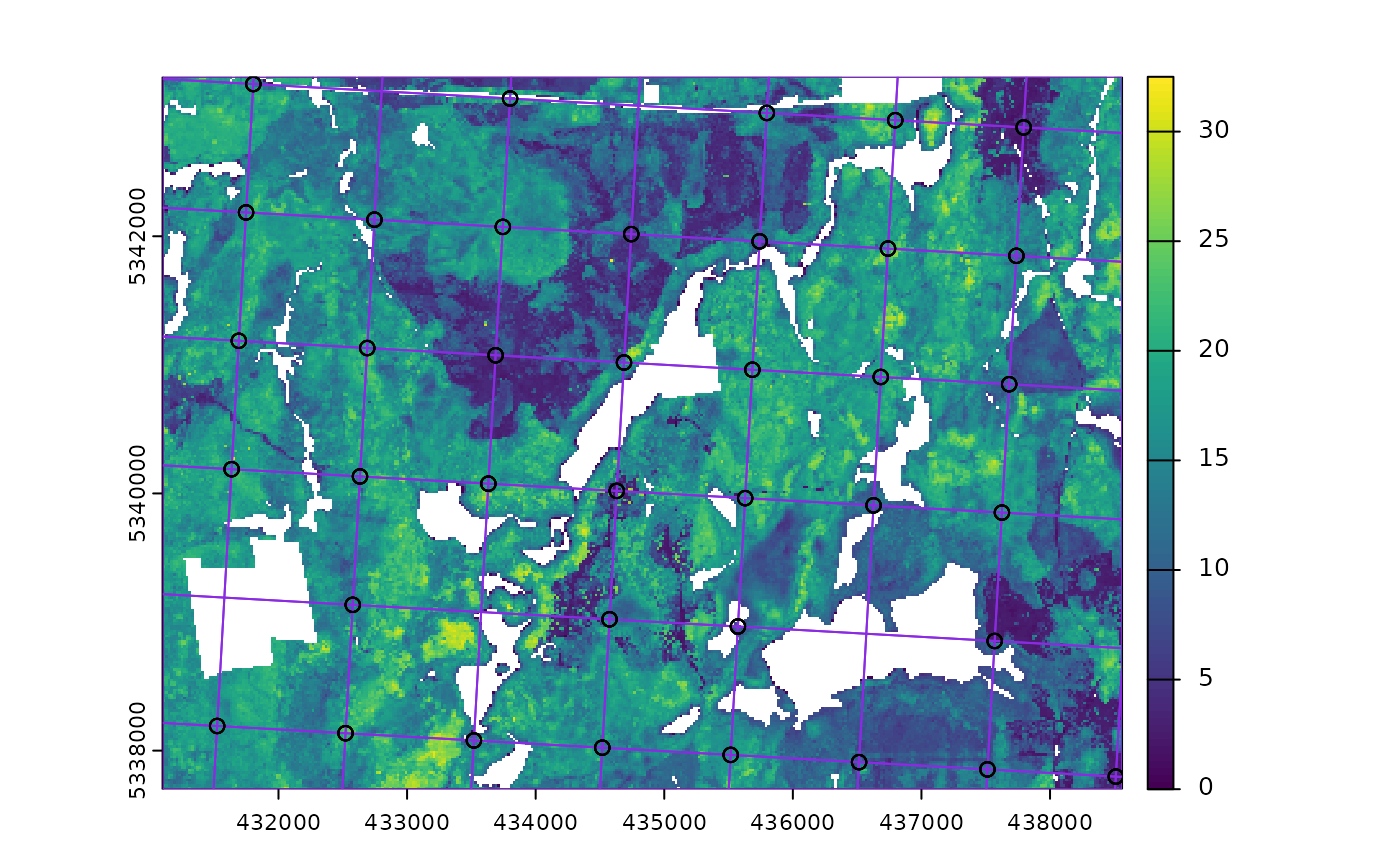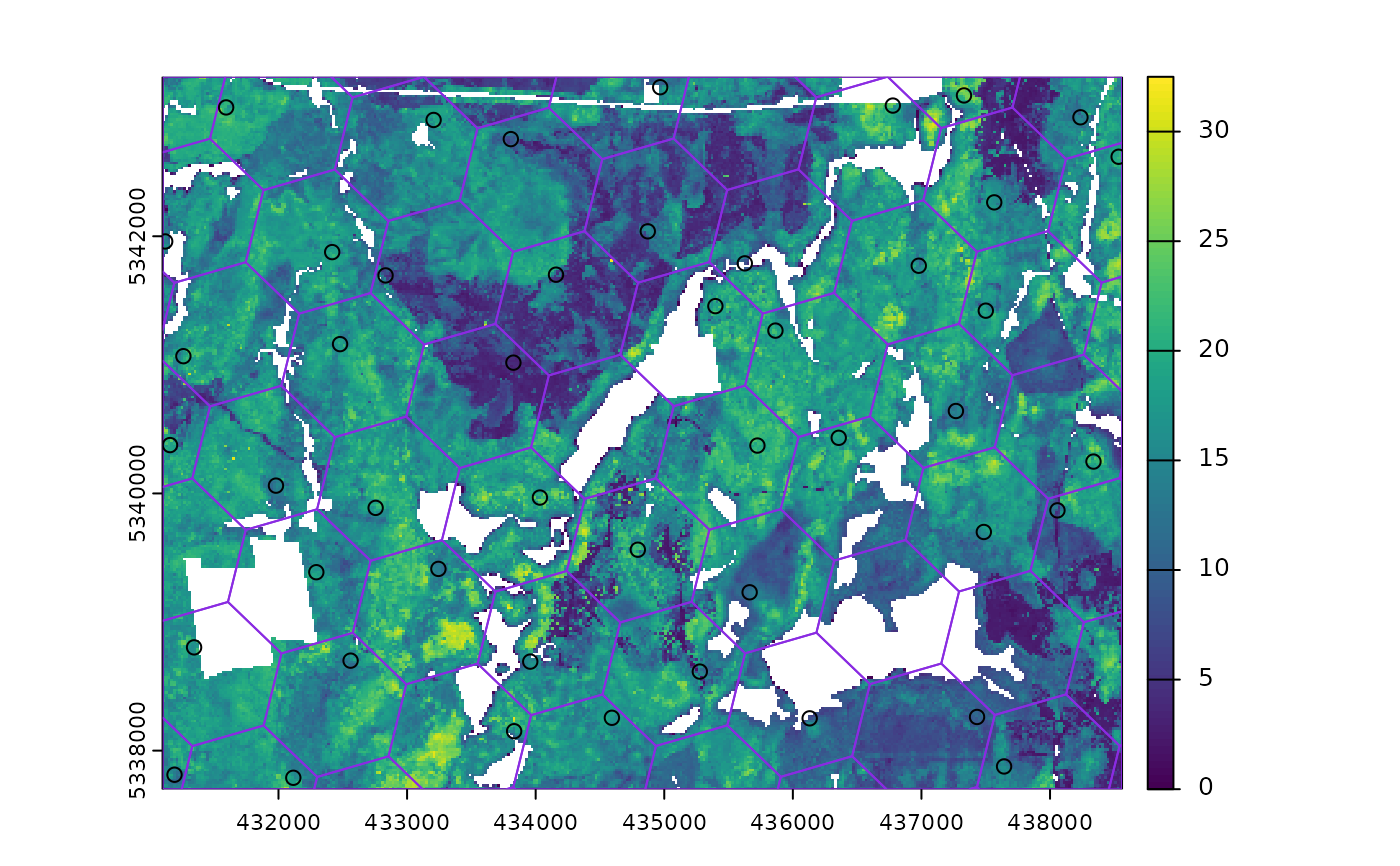Systematic sampling with random start point and translation within a square or hexagonal tessellation.

## Usage

sample_systematic(
raster,
cellsize,
square = TRUE,
location = "centers",
force = FALSE,
access = NULL,
buff_inner = NULL,
buff_outer = NULL,
plot = FALSE,
filename = NULL,
overwrite = FALSE,
details = FALSE,
...
)

## Arguments

raster

spatRaster. Raster used to define extent of fishnet grid.

cellsize

Numeric. Desired cellsize for tessellation.

square

Logical. Tessellation shape. Default is regular square grid, if FALSE hexagons are used.

location

Character. Sample location within tessellation. Default = "centers") returns samples at tessellation centers, "corners" - corners of tessellation are returned, "random" - samples are randomly located within tessellations.

force

Logical. Only applies when location = "random". If TRUE, random samples are forced to fall in areas where raster does not have NA values. This will considerably slow processing.

access

sf. Road access network - must be lines.

buff_inner

Numeric. Inner buffer boundary specifying distance from access where plots cannot be sampled.

buff_outer

Numeric. Outer buffer boundary specifying distance from access where plots can be sampled.

plot

Logical. Plots output strata raster with samples.

filename

Character. Path to write output samples.

overwrite

Logical. Choice to overwrite existing filename if it exists.

details

Logical. If FALSE (default) output is sf object of systematic samples. If TRUE returns a list of sf objects where tessellation is the tessellation grid for sampling, and samples are the systematic samples.

...

Additional arguments for st_make_grid. Options include offset to offset grid by providing lower left coordinates.

## Value

An sf object with sampled points over a tessellation.

## Note

Specifying location = "random" can result in tessellations with no samples. This results from raster have NA values at the random location chosen. Using force = TRUE removes areas of NA from sampling entirely, but considerably slows processing speeds. Thanks to R. Hijmans for help in debugging and providing suggestions for this script.

Other sample functions: sample_ahels(), sample_balanced(), sample_clhs(), sample_existing(), sample_nc(), sample_srs(), sample_strat(), sample_sys_strat()

## Author

Tristan R.H. Goodbody, Lukas Winiwarter

## Examples

#--- Load raster and access files ---#
r <- system.file("extdata", "mraster.tif", package = "sgsR")
mr <- terra::rast(r)
#--- perform grid sampling ---#
sample_systematic(
raster = mr,
cellsize = 1000
)
#> Simple feature collection with 38 features and 0 fields
#> Geometry type: POINT
#> Dimension:     XY
#> Bounding box:  xmin: 431206.1 ymin: 5337760 xmax: 438545 ymax: 5343142
#> Projected CRS: UTM Zone 17, Northern Hemisphere
#> First 10 features:
#>                    geometry
#> 1  POINT (438281.6 5337760)
#> 2  POINT (436061.1 5338024)
#> 3    POINT (437002 5338362)
#> 4  POINT (437942.9 5338701)
#> 5  POINT (432899.7 5337948)
#> 6  POINT (433840.6 5338287)
#> 7  POINT (434781.5 5338626)
#> 8  POINT (435722.4 5338965)
#> 9  POINT (436663.3 5339303)
#> 10 POINT (437604.1 5339642)

sample_systematic(
raster = mr,
cellsize = 1000,
location = "corners",
plot = TRUE
)#> Simple feature collection with 185 features and 0 fields
#> Geometry type: POINT
#> Dimension:     XY
#> Bounding box:  xmin: 431315.3 ymin: 5337714 xmax: 438294.9 ymax: 5343132
#> Projected CRS: UTM Zone 17, Northern Hemisphere
#> First 10 features:
#>                    geometry
#> 1  POINT (435460.6 5337753)
#> 2  POINT (436405.4 5338081)
#> 3  POINT (435460.6 5337753)
#> 4  POINT (437350.1 5338408)
#> 5  POINT (436405.4 5338081)
#> 6  POINT (438294.9 5338736)
#> 7  POINT (437350.1 5338408)
#> 8  POINT (438294.9 5338736)
#> 9  POINT (432298.6 5337714)
#> 10 POINT (433243.3 5338042)

sample_systematic(
raster = mr,
cellsize = 1000,
square = FALSE,
location = "random",
plot = TRUE
)#> Simple feature collection with 45 features and 0 fields
#> Geometry type: POINT
#> Dimension:     XY
#> Bounding box:  xmin: 431131.6 ymin: 5337783 xmax: 438547.8 ymax: 5343238
#> Projected CRS: UTM Zone 17, Northern Hemisphere
#> First 10 features:
#>                    geometry
#> 1  POINT (431131.6 5341862)
#> 2  POINT (431474.3 5342591)
#> 3  POINT (431673.2 5341245)
#> 4    POINT (432697 5341599)
#> 5  POINT (433459.1 5343183)
#> 6  POINT (432191.3 5340911)
#> 7  POINT (433581.8 5341957)
#> 8  POINT (431336.8 5338740)
#> 9  POINT (432109.3 5340180)
#> 10 POINT (433485.6 5340932)